Tamilnadu State Board New Syllabus Samacheer Kalvi 11th Maths Guide Pdf Chapter 11 Integral Calculus Ex 11.12 Text Book Back Questions and Answers, Notes.

## Tamilnadu Samacheer Kalvi 11th Maths Solutions Chapter 11 Integral Calculus Ex 11.12

Integrate the following with respect to x.

Question 1.
(i) $$\sqrt{x^{2}+2 x+10}$$(ii) $$\sqrt{x^{2}-2 x-3}$$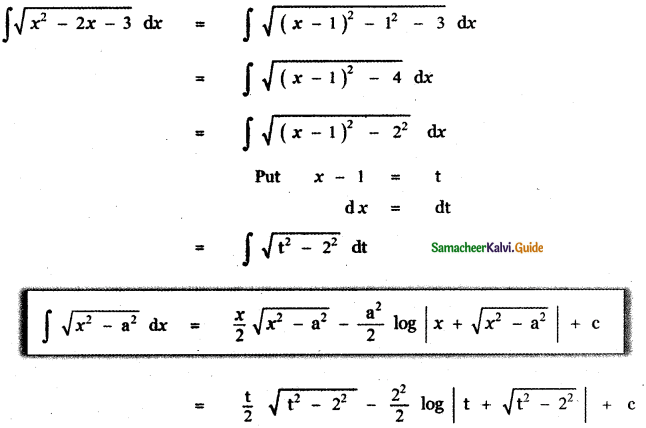(iii) $$\sqrt{(6-x)(x-4)}$$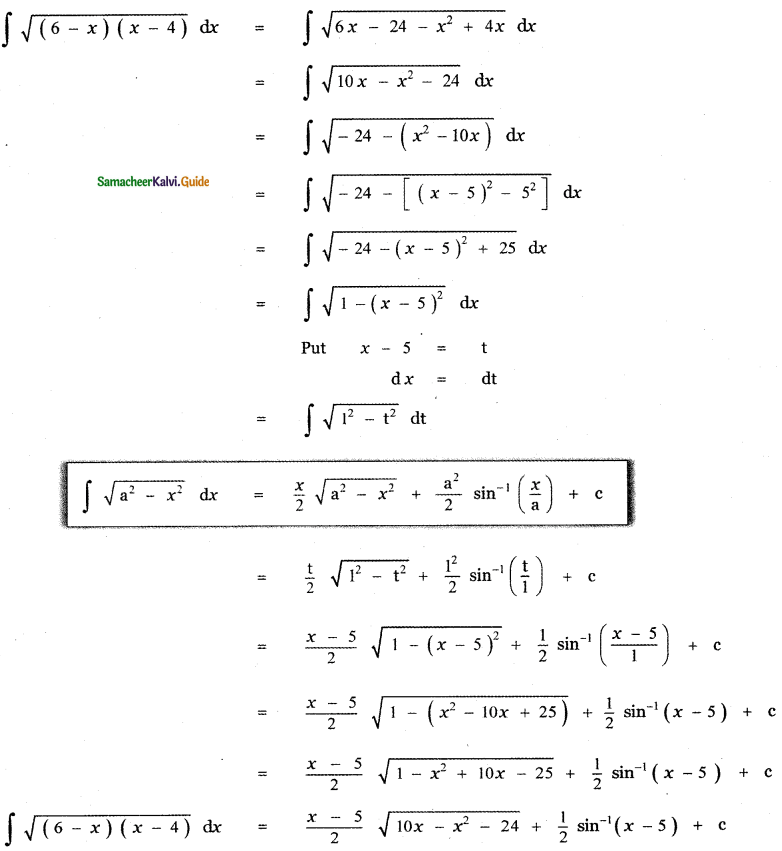Question 2.
(i) $$\sqrt{9-(2 x+5)^{2}}$$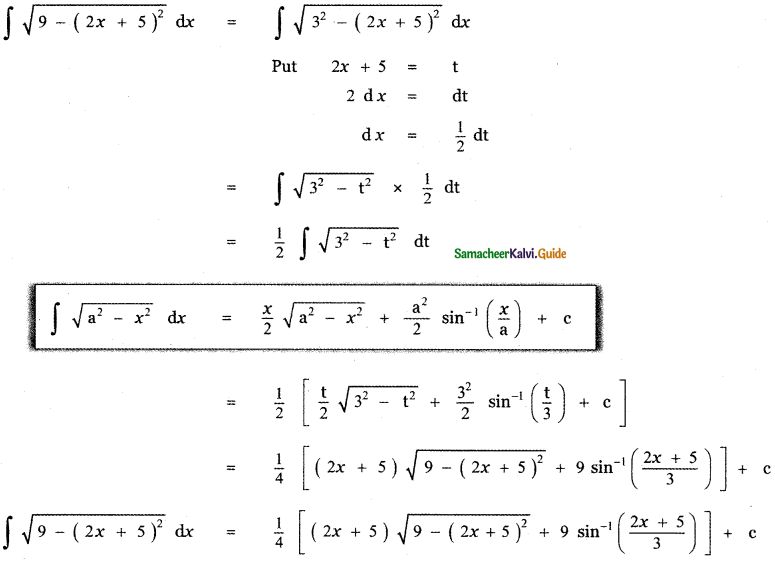(ii) $$\sqrt{81+(2 x+1)^{2}}$$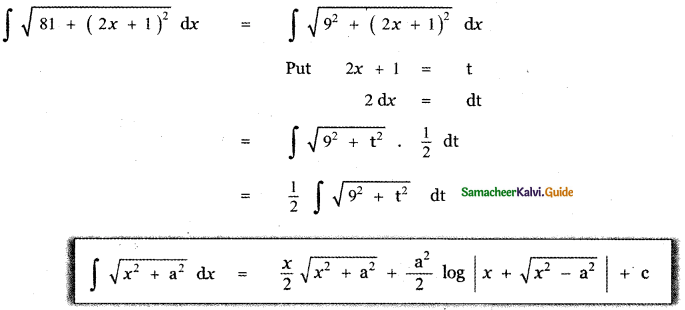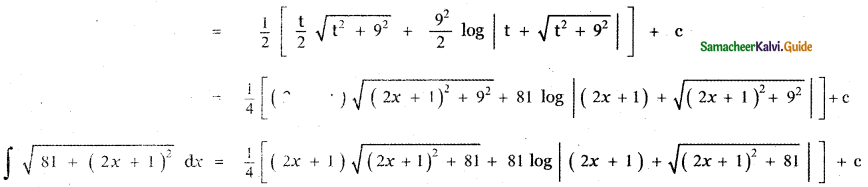(iii) $$\sqrt{(x+1)^{2}-4}$$